# A tutorial on using the suddengains R package

#### 2020-05-22

Please cite this vignette and the R package suddengains as:

citation("suddengains")
#>
#>   Wiedemann, M., Thew, G. R., Stott, R., & Ehlers, A. (2019).
#>   suddengains: An R package to identify sudden gains in longitudinal
#>   data. PLOS ONE. https://doi.org/10.1371/journal.pone.0230276
#>
#> A BibTeX entry for LaTeX users is
#>
#>   @Article{,
#>     author = {Milan Wiedemann and Graham R Thew and Richard Stott and Anke Ehlers},
#>     title = {{suddengains}: {An} {R} package to identify sudden gains in longitudinal data},
#>     journal = {PLOS ONE},
#>     year = {2020},
#>     doi = {10.1371/journal.pone.0230276},
#>     url = {https://github.com/milanwiedemann/suddengains},
#>   }

# Introduction

This vignette shows how the suddengains R package can be used to help with the methods of research studies looking at sudden gains as described by Tang and DeRubeis (1999). More about the theoretical background of sudden gains and why it might be helpful to use this package can be found in our paper Wiedemann et al. (2020). The following vignette illustrates the main functions of the package using the example data set sgdata.

# Data

Below are two interactive tables of depression and rumination scores from the data set (sgdata) that comes with the suddengains package. The data is automatically loaded together with the package when running library(suddengains). Each measured construct contains a baseline measure (s0), twelve weekly measures during therapy (s1 to s12), and two follow-up measures (fu1 and fu2). Note that some values for each measure are missing, shown here as empty cells. For an example of a missing value see bdi_s2 for id = 2 in the table below.

# Preparation of data

## Select cases

The package offers two methods to select cases for the calculation of sudden gains.

1. "pattern": cases providing enough data to apply the Tang and DeRubeis (1999) criteria will be selected
2. "min_sess": cases with a minimum number of available data points (specified in min_sess_num) will be selected

By default the argument return_id_lgl is set to FALSE, which adds a new variable named sg_select at the end of the data frame specified in the data argument. This newly calculated variable sg_select is logical and indicates whether a case is selected (TRUE) or not selected (FALSE) based on the method specified. When the argument return_id_lgl is set to TRUE, only the id variable specified in id_var_name and the new variable sg_select will be returned as the output of this function.

# 1. method = "pattern"
select_cases(data = sgdata,
id_var_name = "id",
sg_var_list = c("bdi_s1", "bdi_s2", "bdi_s3", "bdi_s4",
"bdi_s5", "bdi_s6", "bdi_s7", "bdi_s8",
"bdi_s9", "bdi_s10", "bdi_s11", "bdi_s12"),
method = "pattern",
return_id_lgl = FALSE)

# 2. method = "min_sess"
select_cases(data = sgdata,
id_var_name = "id",
sg_var_list = c("bdi_s1", "bdi_s2", "bdi_s3", "bdi_s4",
"bdi_s5", "bdi_s6", "bdi_s7", "bdi_s8",
"bdi_s9", "bdi_s10", "bdi_s11", "bdi_s12"),
method = "min_sess",
min_sess_num = 9,
return_id_lgl = TRUE)

The following code shows how to select cases based on the "pattern" method and save them as an object called sgdata_select. This function goes through the data and selects all cases with at least one of the following data patterns.

Data pattern xn-2 xn-1 xn xn+1 xn+2 xn+3
1. . x x x x .
2. . x x x . x
3. x . x x x .
4. x . x x . x

Note: xn-2 to xn+3 are consecutive data points of the primary outcome measure. “x” = Present data; “.” = Missing data. “x” represents available data to be examined as a possible pregain session.

sgdata_select <- select_cases(data = sgdata,
id_var_name = "id",
sg_var_list = c("bdi_s1", "bdi_s2", "bdi_s3", "bdi_s4",
"bdi_s5", "bdi_s6", "bdi_s7", "bdi_s8",
"bdi_s9", "bdi_s10", "bdi_s11", "bdi_s12"),
method = "pattern",
return_id_lgl = FALSE) %>%
dplyr::filter(sg_select == TRUE)
#> The method 'pattern' was used to select cases.
#> See help('select_cases') for more information.

# Identification of sudden gains

## Define cut-off for first criterion

This function calculates a cut-off value to use for the first sudden gains criterion based on the Reliable Change Index (RCI, Jacobson and Truax 1991). The following 5 elements are returned by the function

• sd: Standard deviation manually entered using the sd argument or the standard deviation of the values specified in data_sd
• reliability: Reliability of the measure manually specified in reliability or the internal consistency (Cronbach’s alpha) calculated from the item-by-item data specified in data_reliability.
• standard_error_measurement: Standard error of measurement, see formula below:

$\text{standard_error_measurement} = {\text{sd}} \times \sqrt{1-\text{reliability}}$

• standard_error_difference: Standard error of the difference between two test scores, see formula below:

$\text{standard_error_difference} = \sqrt{2\times (\text{standard_error_measurement})^2}$

• reliable_change_value: Value that is considered to reflect reliable change on a measure (Jacobson and Truax 1991). This value is calculated using:

$\text{reliable_change_value} = 1.96 \times \text{standard_error_difference}$

The last element of the list sg_crit1_cutoff can be used as a cut-off value for the first sudden gains criterion.

# Define cut-off value for first SG criterion
# The sd and the reliability are specified manually
define_crit1_cutoff(sd = 10.5,
reliability = 0.931)

# The reliability is specified manually
# The sd gets calculated from variable "bdi_s0" in "sgdata"

sgdata_group$group <- sample(seq(from = 1, to = 2, by = 1), size = 86, replace = TRUE) # Create byperson data set byperson_group <- create_byperson(data = sgdata_group, sg_crit1_cutoff = 7, id_var_name = "id", tx_start_var_name = "bdi_s1", tx_end_var_name = "bdi_s12", sg_var_list = c("bdi_s1", "bdi_s2", "bdi_s3", "bdi_s4", "bdi_s5", "bdi_s6", "bdi_s7", "bdi_s8", "bdi_s9", "bdi_s10", "bdi_s11", "bdi_s12"), sg_measure_name = "bdi", multiple_sg_select = "first") #> First, second, and third sudden gains criteria were applied. #> The critical value for the third criterion was adjusted for missingness. #> The first gain/loss was selected in case of multiple gains/losses. byperson_group_select <- select_cases(data = byperson_group, id_var_name = "id", sg_var_list = c("bdi_s1", "bdi_s2", "bdi_s3", "bdi_s4", "bdi_s5", "bdi_s6", "bdi_s7", "bdi_s8", "bdi_s9", "bdi_s10", "bdi_s11", "bdi_s12"), method = "pattern", return_id_lgl = FALSE) %>% dplyr::filter(sg_select == TRUE) #> The method 'pattern' was used to select cases. #> See help('select_cases') for more information. The data frame sgdata_group can now be used to illustrate the plotting function for different groups. This function works identically to the plot function for a single group. Further arguments are used to specify the colours to be used for the different groups. plot_byperson_group <- plot_sg(data = byperson_group_select, id_var_name = "id", tx_start_var_name = "bdi_s1", tx_end_var_name = "bdi_s12", sg_pre_post_var_list = c("sg_bdi_2n", "sg_bdi_1n", "sg_bdi_n", "sg_bdi_n1", "sg_bdi_n2", "sg_bdi_n3"), group_var_name = "group", group_levels = c(1, 2), group_labels = c("Treatment A", "Treatment B"), group_title = NULL, colour_group = "viridis", viridis_option = "B", viridis_begin = 0.2, viridis_end = 0.8, apaish = TRUE, ylab = "BDI", xlab = "Session") The plot below shows the average gain for each group. plot_byperson_group #> Warning: Removed 402 rows containing non-finite values (stat_summary). #> Warning: Removed 402 rows containing non-finite values (stat_summary). #> Warning: Removed 106 rows containing non-finite values (stat_summary). #> Warning: Removed 304 rows containing non-finite values (stat_summary). #> Warning: Removed 98 rows containing non-finite values (stat_summary).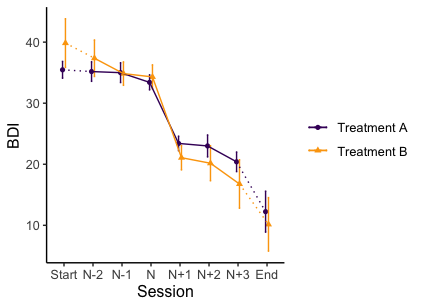## Plot individual trajectories The function plot_sg_trajectories() can plot individual trajectories of the cases in the data set. It is possible to select specific IDs for plotting using the select_id_list argument, or a number of random IDs using the sample_n argument. Below, case IDs 2, 4, 5 and 9 are selected. plot_trajectories_1 <- sgdata %>% plot_sg_trajectories(id_var = "id", select_id_list = c("2", "4", "5", "9"), var_list = c("bdi_s1", "bdi_s2", "bdi_s3", "bdi_s4", "bdi_s5", "bdi_s6", "bdi_s7", "bdi_s8", "bdi_s9", "bdi_s10", "bdi_s11", "bdi_s12"), show_id = TRUE, id_label_size = 4, label.padding = 0.2, show_legend = FALSE, colour = "viridis", viridis_option = "D", viridis_begin = 0, viridis_end = 0.8, connect_missing = FALSE, scale_x_num = TRUE, scale_x_num_start = 1, apaish = TRUE, xlab = "Session", ylab = "BDI") plot_trajectories_1 #> Warning: Removed 3 rows containing missing values (geom_point). #> Warning: Removed 3 rows containing missing values (geom_label_repel).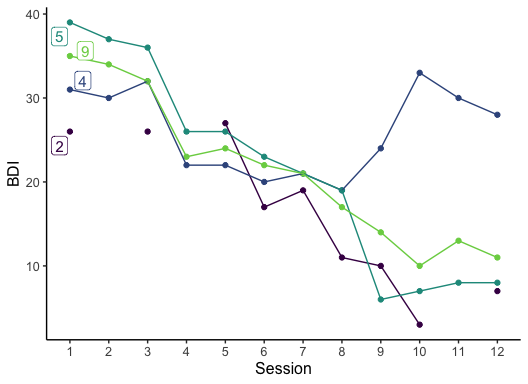This function can also be combined with a filter function to explore specific groups of sudden gains cases, for example (1) all cases with a sudden gain at session 3, or (2) three randomly selected (select_n = 3) cases who experienced more than one sudden gain (dplyr::filter(sg_freq_byperson > 1). # 1. Create plot including all cases with a sudden gain at session 3 plot_trajectories_2 <- bysg %>% dplyr::filter(sg_session_n == 3) %>% plot_sg_trajectories(id_var = "id_sg", var_list = c("bdi_s1", "bdi_s2", "bdi_s3", "bdi_s4", "bdi_s5", "bdi_s6", "bdi_s7", "bdi_s8", "bdi_s9", "bdi_s10", "bdi_s11", "bdi_s12"), show_id = FALSE, id_label_size = 4, label.padding = 0.2, show_legend = TRUE, colour = "viridis", viridis_option = "D", viridis_begin = 0, viridis_end = 0.8, connect_missing = TRUE, scale_x_num = TRUE, scale_x_num_start = 1, apaish = TRUE, xlab = "Session", ylab = "BDI") # 1. Show all cases with a sudden gain at session 3 plot_trajectories_2 #> Warning: Removed 4 rows containing missing values (geom_point).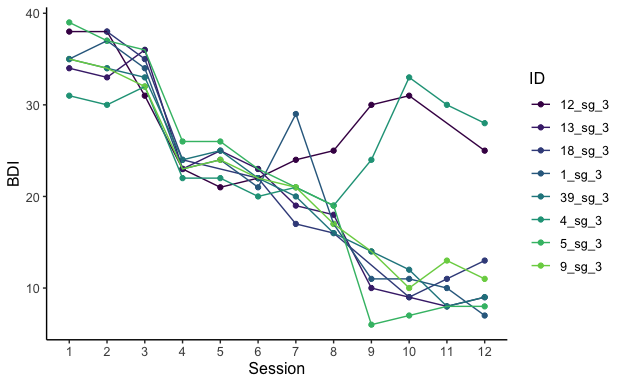# 2. Create plot including 3 randomly selected (select_n = 3) cases who experienced # more than 1 gain (dplyr::filter(sg_freq_byperson > 1)) plot_trajectories_3 <- byperson_first %>% dplyr::filter(sg_freq_byperson > 1) %>% plot_sg_trajectories(id_var = "id_sg", var_list = c("bdi_s1", "bdi_s2", "bdi_s3", "bdi_s4", "bdi_s5", "bdi_s6", "bdi_s7", "bdi_s8", "bdi_s9", "bdi_s10", "bdi_s11", "bdi_s12"), select_n = 3, show_id = FALSE, id_label_size = 4, label.padding = 0.2, show_legend = TRUE, colour = "viridis", viridis_option = "D", viridis_begin = 0, viridis_end = 0.8, connect_missing = TRUE, scale_x_num = TRUE, scale_x_num_start = 1, apaish = TRUE, xlab = "Session", ylab = "BDI") # 2. Show 3 cases (select_n = 3) with more than 1 gain (dplyr::filter(sg_freq_byperson > 1)) plot_trajectories_3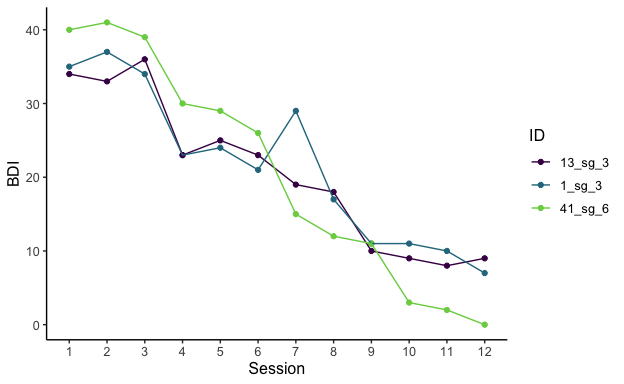# Summarise descriptive statistics ## Count between-session intervals The count_intervals function provides a summary of how many between-session intervals were and were not analysed for sudden gains. For more information see the help file for this function, help(count_intervals). Here we see code to count only the intervals of the data that was selected for the sudden gains study in the above code using sgdata_select. • total_between_sess_intervals: The total number of between-session intervals present in the data set, here: sgdata_select. • total_between_sess_intervals_sg: The total number of gain intervals (i.e. sudden gains) present in the data set. By default the first-to-second and penultimate-to-last intervals are not included here. If identify_sg_1to2 is set to TRUE the first-to-second intervals will be included. • available_between_sess_intervals_sg: The total number of between-session intervals that could feasibly be analysed for sudden gains. • not_available_between_sess_intervals_sg: The total number of between-session intervals that could not be analysed for sudden gains (due to missing data). count_intervals(data = sgdata_select, id_var_name = "id", sg_var_list = c("bdi_s1", "bdi_s2", "bdi_s3", "bdi_s4", "bdi_s5", "bdi_s6", "bdi_s7", "bdi_s8", "bdi_s9", "bdi_s10", "bdi_s11", "bdi_s12"), identify_sg_1to2 = FALSE) #>$total_between_sess_intervals
#>  429
#>
#> $total_between_sess_intervals_sg #>  351 #> #>$available_between_sess_intervals_sg
#>  298
#>
#> $not_available_between_sess_intervals_sg #>  53 The plot_sg_intervals() can be used to visualise the available data for each time point as well as the percentage of session to session intervals that are analysed for potential sudden gains. plot_sg_intervals(data = sgdata_select, id_var_name = "id", sg_var_list = c("bdi_s1", "bdi_s2", "bdi_s3", "bdi_s4", "bdi_s5", "bdi_s6", "bdi_s7", "bdi_s8", "bdi_s9", "bdi_s10", "bdi_s11", "bdi_s12"))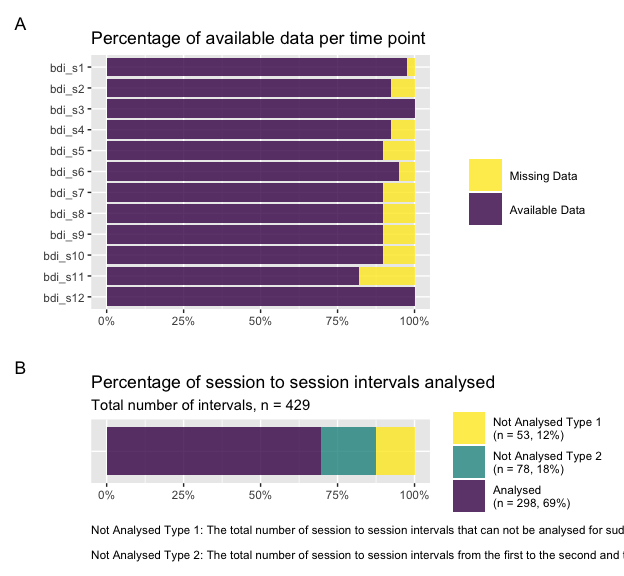## Descriptive statistics of sudden gains The describe_sg() function provides descriptive statistics about the sudden gains based on the variables from the bysg or byperson datasets. The descriptives (e.g. “sg_pct”, the percentage of cases with sudden gains in the specified data set) are always in relation to the input data and therefore will vary depending on whether the structure of the data set is bysg or byperson. # Describe bysg dataset ---- describe_sg(data = bysg, sg_data_structure = "bysg") #>$total_n
#>  24
#>
#> $sg_total_n #>  24 #> #>$sg_pct
#>  100
#>
#> $sg_multiple_pct #>  70.83 #> #>$sg_reversal_n
#>  4
#>
#> $sg_reversal_pct #>  16.67 #> #>$sg_magnitude_m
#>  11
#>
#> $sg_magnitude_sd #>  3.43 # Describe byperson dataset ---- describe_sg(data = byperson_first, sg_data_structure = "byperson") #>$total_n
#>  43
#>
#> $sg_total_n #>  24 #> #>$sg_n
#>  15
#>
#> $sg_pct #>  34.88 #> #>$sg_multiple_n
#>  8
#>
#> $sg_multiple_pct #>  18.6 #> #>$sg_reversal_n
#>  3
#>
#> $sg_reversal_pct #>  20 #> #>$sg_magnitude_m
#>  11.4
#>
#> \$sg_magnitude_sd
#>  3.98